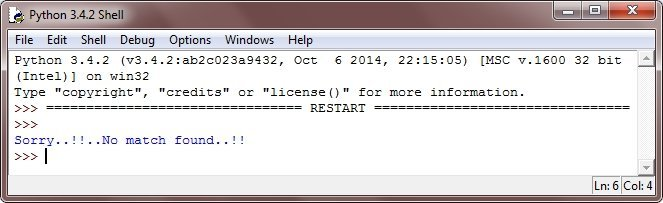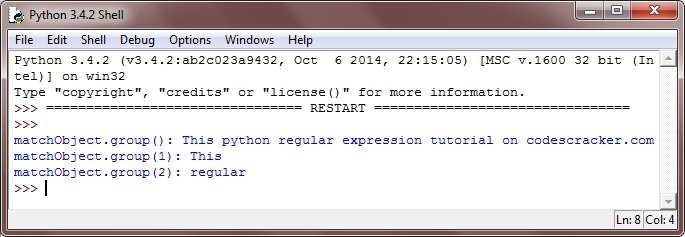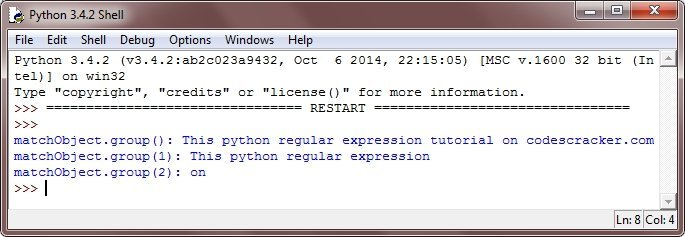# Python Regular Expression

A regular expression in python, is simply a special sequence of characters, helps you to match the other set of strings, using specialized syntax, in a pattern.

A regular expression is a special sequence of characters that helps you to match or find the other (or set of) strings, using a specialized syntax held in a pattern. Regular expressions are widely used in UNIX.

The match() function in python, attempts to match RE pattern to the string with an optional flag. Here is the general form to use match() function in python:

`re.match(pattern, string, flags=0)`

Here, pattern is that regular expression which is to be matched, string is the string, which would be searched to match the pattern at the beginning of the string, and then flags are the modifiers.

The match() function just returns the matched object on success, otherwise none. You can use groups() function of match object to get the matched expression.

## Python Regular Expression Example

Here is an example program, illustrates the concept and uses of regular expression in python:

```# Python Regular Expression - Example Program

import re

str = "This python regular expression tutorial on codescracker.com"

matchObject = re.match( r'(.*) are (.*?) .*', str, re.M|re.I)

if matchObject:
print("matchObject.group():", matchObject.group())
print("matchObject.group(1):", matchObject.group(1))
print("matchObject.group(2):", matchObject.group(2))

else:
print("Sorry..!!..No match found..!!")```

Here is the sample output of the above python program:Let's modify the above python program with this:

```# Python Regular Expression - Example Program

import re

str = "This python regular expression tutorial on codescracker.com"

matchObject = re.match( r'(.*) python (.*?) .*', str, re.M|re.I)

if matchObject:
print("matchObject.group():", matchObject.group())
print("matchObject.group(1):", matchObject.group(1))
print("matchObject.group(2):", matchObject.group(2))

else:
print("Sorry..!!..No match found..!!")```

Here is the sample output produced by the above python program:Let's again modify the above python program with this:

```# Python Regular Expression - Example Program

import re

str = "This python regular expression tutorial on codescracker.com"

matchObject = re.match( r'(.*) tutorial (.*?) .*', str, re.M|re.I)

if matchObject:
print("matchObject.group():", matchObject.group())
print("matchObject.group(1):", matchObject.group(1))
print("matchObject.group(2):", matchObject.group(2))

else:
print("Sorry..!!..No match found..!!")```

Below is the output produced by the above python program:Tools
Calculator